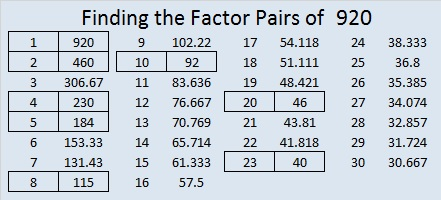# 920 Witches’ Cauldron

“Double, double toil and trouble;
Fire burn, and caldron bubble.”

What besides “eye of newt” goes in witches’ cauldrons? The list includes some horrifying ingredients that you can read here from one scene from Shakeaspeare’s play, MacBeth.

Instead of putting “Eye of newt, and toe of frog, Wool of bat, and tongue of dog” and so forth in today’s Halloween cauldron puzzle, I just put a bunch of asterisks.Print the puzzles or type the solution on this excel file: 10-factors-914-922

“Double, double toil and trouble;
Fire burn, and caldron bubble.”

Double 115 is 230.

Double 230 is 460.

Double 460 is 920, today’s post number.

920 is the hypotenuse of a Pythagorean triple:
552-736-920 which is (3-4-5) times 184.

920 is palindrome 767 in BASE 11 because 7(121) + 6(11) + 7(1) = 920

• 920 is a composite number.
• Prime factorization: 920 = 2 × 2 × 2 × 5 × 23, which can be written 920 = 2³ × 5 × 23
• The exponents in the prime factorization are 3, 1, and 1. Adding one to each and multiplying we get (3 + 1)(1 + 1)(1 + 1) = 4 × 2 × 2 = 16. Therefore 920 has exactly 16 factors.
• Factors of 920: 1, 2, 4, 5, 8, 10, 20, 23, 40, 46, 92, 115, 184, 230, 460, 920
• Factor pairs: 920 = 1 × 920, 2 × 460, 4 × 230, 5 × 184, 8 × 115, 10 × 92, 20 × 46, or 23 × 40
• Taking the factor pair with the largest square number factor, we get √920 = (√4)(√230) = 2√230 ≈ 20.331501776.